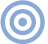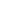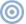Features

Quality Lies in the Details SIDEBAR 4: Capacitors & Capacitance

SIDEBAR 4: Capacitors & Capacitance
Capacitance is associated with "capacitive reactance," which can be thought of as frequency-dependent resistance. As frequency increases, the capacitance produces less and less "reactance," or resistance to current flow. The higher the frequency, the lower the opposition to current flow produced by the capacitance.

A cable's capacitive reactance can be thought of as a resistor in parallel with the load. As frequency increases (and the capacitive reactance decreases), more and more of the drive signal is dropped across the cable's capacitance, and less across the load (the component we're driving). This is why cable capacitance should be kept low; the frequency at which rolloff begins is higher for lower-capacitance cable. To calculate the approximate -3dB low-pass point for a cable/source component combination, use the formula f = 1/(2PiRC), where Pi = 3.142, C is the value of the shunt capacitor in farads, and R is the resistance in ohms of the source component's output resistance.

With a high source impedance of 2k ohms (2000 ohms) and a cable capacitance of 3nF (3000pF), the -3dB high-frequency rolloff point will be 26kHz, resulting in an audibly dulled top octave. With a more typical source impedance of 100 ohms, the -3dB point with the same cable moves up to a completely innocuous 530kHz.—Robert Harley & John AtkinsonARTICLE CONTENTSX